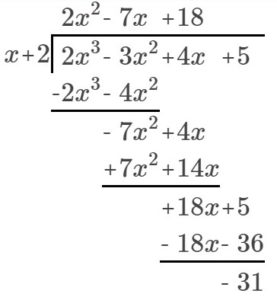## Divide Polynomials Part II

### Learning Outcome

• Multiply and divide polynomials

As we have seen, long division of polynomials can involve many steps and be quite cumbersome. Synthetic division is a shorthand method of dividing polynomials for the special case of dividing by a polynomial whose leading coefficient is $1$.

### Synthetic Division

Synthetic division is a shortcut that can be used when the divisor is a binomial in the form  $x–k$, for a real number $k$. In synthetic division, only the coefficients are used in the division process.

To illustrate the process, divide $2{x}^{3}-3{x}^{2}+4x+5$ by $x+2$ using the long division algorithm.There is a lot of repetition in this process. If we do not write the variables but, instead, line up their coefficients in columns under the division sign, we already have a simpler version of the entire problem.Synthetic division carries this simplification even a few more steps. Collapse the table by moving each of the rows up to fill any vacant spots. Also, instead of dividing by $2$, as we would in division of whole numbers, then multiplying and subtracting the middle product, we change the sign of the “divisor” to $–2$, multiply and add. The process starts by bringing down the leading coefficient.We then multiply it by the “divisor” and add, repeating this process column by column, until there are no entries left. The bottom row represents the coefficients of the quotient; the last entry of the bottom row is the remainder. In this case, the quotient is $2x{^2} -7x+18$ and the remainder is $–31$. The process will be made more clear in the following example.

### Example

Use synthetic division to divide $5{x}^{2}-3x - 36$ by $x - 3$.

Analysis of the solution

It is important to note that the result, $5x+12$, of $5{x}^{2}-3x - 36\div{x-3}$ is one degree less than $5{x}^{2}-3x - 36$. Why is that? Think about how you would have solved this using long division. The first thing you would ask yourself is how many x’s are in $5x^2$?

$x-3\overline{)5{x}^{2}-3x - 36}$

To get a result of $5x^2$, you need to multiply $x$ by $5x$. The next step in long division is to subtract this result from $5x^2$. This leaves us with no $x^2$ term in the result.

Reflect on this idea – if you multiply two polynomials and get a result whose degree is $2$, what are the possible degrees of the two polynomials that were multiplied? Write your ideas in the box below before looking at the discussion.

In the next video example, you will see another example of using synthetic division for division of a degree two polynomial by a degree one binomial.

### How To: Given two polynomials, use synthetic division to divide

1. Write k for the divisor.
2. Write the coefficients of the dividend.
3. Bring the lead coefficient down.
4. Multiply the lead coefficient by k. Write the product in the next column.
5. Add the terms of the second column.
6. Multiply the result by k. Write the product in the next column.
7. Repeat steps $5$ and $6$ for the remaining columns.
8. Use the bottom numbers to write the quotient. The number in the last column is the remainder and has degree $0$. The next number from the right has degree $1$, and the next number from the right has degree $2$, and so on.

In the next example, we will use synthetic division to divide a third-degree polynomial.

### Example

Use synthetic division to divide $4{x}^{3}+10{x}^{2}-6x - 20$ by $x+2$.

In the next example, we will show division of a fourth degree polynomial by a binomial.  Note how there is no x term in the fourth degree polynomial, so we need to use a placeholder of 0 to ensure proper alignment of terms.

### Example

Use synthetic division to divide $-9{x}^{4}+10{x}^{3}+7{x}^{2}-6$ by $x - 1$.

In our last video example, we show another example of how to use synthetic division to divide a degree three polynomial by a degree one binomial.